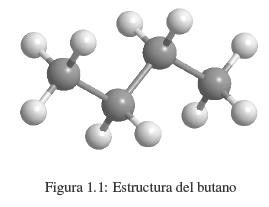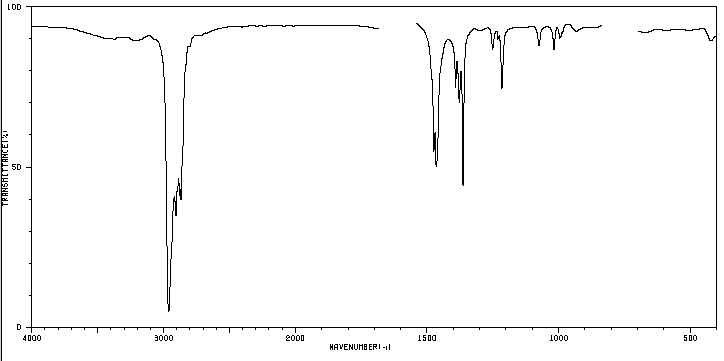## Molecular FormulasCurrently, more than 30 million chemical compounds are known, of which 1 million are inorganic and the rest are organic. Chemists determine the physical and chemical properties of these substances (melting and boiling points, solubility, density...). However, the information most coveted by the chemist is the determination of the structure of the compound, what type of atoms make it up and how these atoms are bonded.
We know that a substance like butane is made up of carbon and hydrogen. Each molecule contains 4 carbon atoms and 10 hydrogen atoms, which is represented by the formula C 4 H 10 , called the molecular formula. We also know that carbon atoms are linked to form a linear chain. 3 hydrogens are attached to the first carbon, 2 hydrogens to the second carbon, 2 hydrogens to the third carbon, and the last 3 hydrogens to the fourth carbon.

It consists of experimentally determining the percentage composition (by mass) of a substance. Thus, for a compound with molecular formula C 4 H 10 the percentage composition is: 82.66% C and 17.34% H.
The elemental analysis of a compound is carried out in an apparatus, called an analyzer, where a weighed sample is burned with great precision (about 5 mg). Carbon is transformed into CO 2 , hydrogen into H 2 O and nitrogen into N 2 , determined by gas chromatography. Oxygen is not usually analyzed and is determined by difference.

From the centesimal composition of a chemical compound we can determine its empirical formula. The molecular formula is obtained from the empirical one using the molecular mass of the compound, which can be determined by mass spectrometry.

Determination of the empirical and molecular formula of cortisone
The percentage composition of cortisone is: 69.96% of C; 7.83% H and 22.21% O. Determine the empirical formula. Knowing that the molecular mass is 360.43, obtain the molecular formula.

The unsaturation index of a molecule is defined as the sum of the number of bonds $\pi$ and the number of cycles present. Alkanes are saturated hydrocarbons as they do not contain $\pi$ bonds or cycles and have the molecular formula $C_nH_{2n+2}$. To calculate the unsaturation index of a compound, we only have to compare the number of hydrogens it has with those it would have if it were an alkane with the same number of carbons. This hydrogen difference divided by 2 gives us the unsaturation index.

Alkenes are compounds that contain carbon-carbon double bonds. Alkenes respond to the molecular formula $C_nH_{2n}$. For the same number of carbons they have two less hydrogens than an alkane, they are said to be unsaturated compounds (they have unsaturation).

An organic compound whose percentage composition is 83.63% carbon and 16.37% hydrogen with a molecular mass of 86.11, presents the following IR spectrum.Determine the structure of the compound.